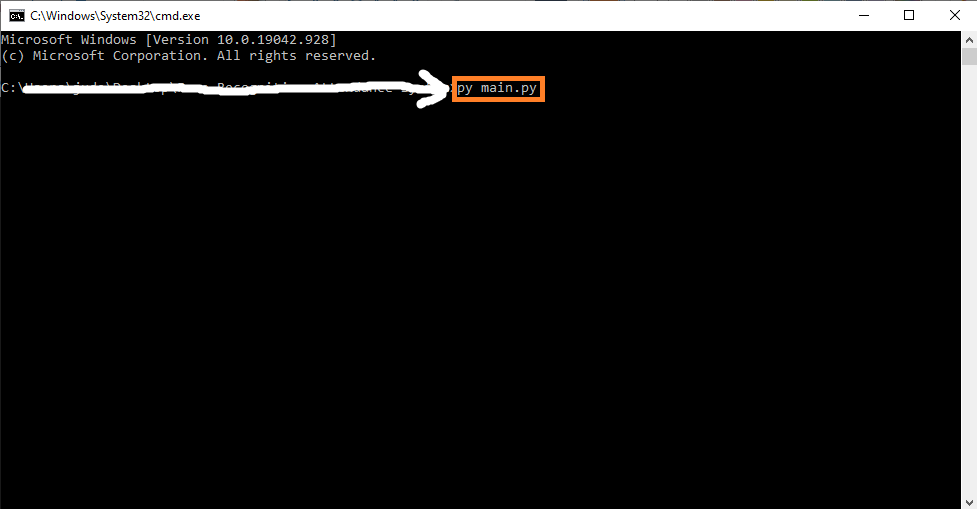# Angle Finder OpenCV Python With Source Code

## Angle Finder OpenCV Python With Source Code

The Angle Finder OpenCV Python was developed using Python OpenCV, In this Python Project With Source Code we will learn on How To Create The Project Using OpenCV Python. We will first define two lines using mouse clicks and then find the angle between these lines using simple mathematics.

## What is OpenCV?

OpenCV is short for Open Source Computer Vision. Intuitively by the name, it is an open-source Computer Vision and Machine Learning library. This library is capable of processing real-time image and video while also boasting analytical capabilities. It supports the Deep Learning frameworks.

By the way if you are new to python programming and you don’t know what would be the the Python IDE to use, I have here a list of Best Python IDE for Windows, Linux, Mac OS that will suit for you. I also have here How to Download and Install Latest Version of Python on Windows.

To start executing Angle Finder OpenCV Python With Source Code, make sure that you have installed Python 3.9 and PyCharm in your computer.

## Angle Finder OpenCV Python With Source Code : Steps on how to run the project

Time needed: 5 minutes

These are the steps on how to run Angle Finder OpenCV Python With Source Code

First, download the given source code below and unzip the source code.• Step 2: Import the project to your PyCharm IDE.• Step 3: Run the project.

last, run the project with the command “py main.py”## Installed Libraries

```import cv2
import math```

## Complete Source Code

```import cv2
import math

path = 'angle.jpg'
pointsList = []

def mousePoints(event,x,y,flags,params):
if event == cv2.EVENT_LBUTTONDOWN:
size = len(pointsList)
if size != 0 and size % 3 != 0:
cv2.line(img,tuple(pointsList[round((size-1)/3)*3]),(x,y),(0,0,255),2)
cv2.circle(img,(x,y),5,(0,0,255),cv2.FILLED)
pointsList.append([x,y])

return (pt2-pt1)/(pt2-pt1)

def getAngle(pointsList):
pt1, pt2, pt3 = pointsList[-3:]
angR = math.atan((m2-m1)/(1+(m2*m1)))
angD = round(math.degrees(angR))

cv2.putText(img,str(angD),(pt1-40,pt1-20),cv2.FONT_HERSHEY_COMPLEX,
1.5,(0,0,255),2)

while True:

if len(pointsList) % 3 == 0 and len(pointsList) !=0:
getAngle(pointsList)

cv2.imshow('Image',img)
cv2.setMouseCallback('Image',mousePoints)
if cv2.waitKey(1) & 0xFF == ord('q'):
pointsList = []
```